Teaching support from the UK’s largest provider of in-school maths tuitionone to one lessonsschools supported

Online one to one tuition designed to boost confidence, engagement and verbal fluency

See it in action

Hundreds of FREE online maths resources!

Daily activities, ready-to-go lesson slides, SATs revision packs, video CPD and more!Explore all resources
Key Stage 2 (Other Topics)

Online one to one tuition designed to boost confidence, engagement and verbal fluency

See it in action

# How to Teach Algebra KS2: Maths Bootcamp 

Want to know how to teach algebra to KS2 pupils? You’ve hit the jackpot with our Algebra Intervention Bootcamp!

The bootcamp series is designed to support Year 5 & 6 teachers, Maths coordinators, intervention leaders, and SATS booster group leaders achieve age related expectations with pupils who need that extra intervention.

By popular demand, our next bootcamp post specifically looks at algebra! This post will help school leaders, Maths coordinators and upper Key Stage 2 teachers diagnose where pupils are struggling in algebra, the ‘nuts and bolts’ of the topic.

We then track back to different stages of understanding, before giving strategies that can be used with whole classes, booster groups or alongside 1-to-1 interventions, such as those provided by Third Space Learning.

#### Each post follows a similar structure:

1. First, you’ll diagnose where pupils are struggling with ‘the nuts and bolts’ of that area of Mathematics from the National Curriculum.
2. Next, you’ll track back to the different stages of understanding and examine what the misconception might be in detail.
3. Finally, we’ll give you strategies that can be used with whole classes, booster groups or alongside 1-to-1 interventions.

#### In this post on algebra, we help you solve problems such as:

• Pupils seem to need guiding through every stage of every algebra question.
• Pupils don’t ‘see’ some questions as algebra when it is in another context.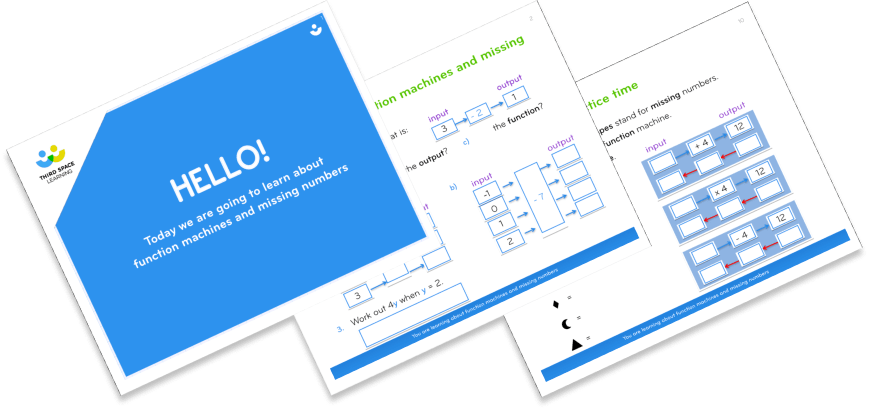Algebra Lessons Resource Pack

Plug gaps and help conquer common upper KS2 misconceptions in algebraDownload Now

#### DIAGNOSIS: what are pupils’ key misconceptions in algebra?

From the below pupils, find the best fit for those you are looking at. Then use this to guide where in the post to read for support:

Sienna is adept at most areas of Maths. Algebra is no different, however she does need to ‘brush up’ on her ability to make connections with other areas of Maths that would help her solve algebraic equations. Stage: SECURING.

Xue has always struggled with missing number equations. She can cope with them when learnt by rote but, if the steps to follow don’t work out the problem, she becomes flustered and doesn’t know what to do next. Similarly, in  Algebra she can tell you what the perimeter of a rectangle is when representing numbers with letters, but can’t find a possible answer for 2x + y = 12. Stage: EMERGING.

Grant hasn’t had a lot of experience with missing numbers and this shows when tackling algebraic equations. He can calculate simple equations but finds it hard when in the context of measures, etc. Stage: DEVELOPING.

Benjamin is comfortable when he knows exactly what to do and has time to do it. Algebra seems like a foreign language to him and he puts seemingly random numbers anywhere, usually by adding or subtracting digits that he can see in the equation. When presented with missing number calculations, he doesn’t understand when or why to use the inverse or commutative properties of the numbers. Stage: PRE BOOSTER.

Benjamin’s needs are beyond the scope of a booster group or this post. He needs specifically targeted 1-to-1 support such as that provided by Third Space Learning to catch him up to a point where booster intervention could be considered. Read my review of Third Space Learning’s 1-to-1 Maths programme if you’re interested in how Third Space can boost attainment and confidence in your school.

#### Misconceptions & strategies for ‘Emerging’ in algebra

Remember, pupils who have not experienced the 2014 National Curriculum from the start of their school lives may have insufficient experience with missing numbers to make links between them and algebra. This means they may not have a strong foundation for further exploration.

Therefore, to help pupils like Xue become more confident with algebra, they need to gain a better understanding of missing numbers.

##### First steps – understanding equals means ‘same as’

Start by giving Xue and the other pupils simple missing number problems such as 62 + [ ] = 112 being sure to include examples with missing numbers at the start: [ ] + 62 = 112. Re-introduce the equals sign as ‘the same as’, so in the first example, 62 add ‘something’ is the same as 112.

To ensure pupils understand what is being asked in any missing number questions, show them a pair of balancing scales commonly used in EYFS and KS1 to compare amounts. Then blu-tac the equals sign (‘the same as’) to the middle of the balance and discuss how pupils need to make both sides the same.

You can then place a weight representing 112 on one side and a second weight representing 62 on the other. This means you can let the pupils discuss what this means, ergo that 112 is heavier, so we need to add more to 62 to make them the same.

Now you can move past the scale analogy and give the pupils plenty of opportunity with simple calculations spanning all 4 operators, such as [ ] x 12 = 96. Ask the pupils why we don’t work out 12 x 96, i.e. because x lots of 12 is the same as 96 and if we do 12 x 96 we will have more than 96.”

Then look at the inverse: [ ] ÷ 12 = 8 and ask the pupils what they know, the answer should be that we have to start with a larger number than 12 because we have 8 whole ones on the other side of the equals sign – so something grouped into 12’s is the same as 8.

The pupils will either see that they can do 12 x 8 or 8 x 12 OR need to get counters out and put them into groups of 12. If they do, let them just for this example, so that afterwards you can make links between arrays and division and using the inverse to help – i.e. “if 96 is split into 12 groups of 8, we could have just put those 12 groups of 8 together to find the number, 96”.

Once the pupils firmly understand the equals sign means the same as and they have a basic grasp of using the inverse to help them solve missing number problems, you are ready to move on to algebraic equations.

#### Misconceptions and strategies for ‘Developing’ in algebra

Now it is time to recap basic algebra to ensure Xue can move along at the same pace as pupils like Grant, who needs to ensure he fully understands the basics in order to tackle more complex algebra.

##### Next steps – practising basic algebra

Show the pupils this and ask what is the value of c:

a + a + a = 21
a + a + b = 25
b + b + c = 32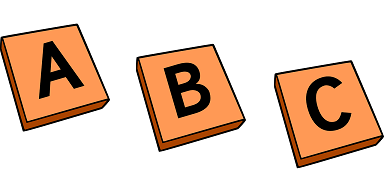Ask the pupils how we can work this out, directing them to look at what they know. In other words, that the second and third lines contain incomplete information because we don’t know the value of ‘a’, ‘b’, or ‘c’, so we cannot work out values from these. Whereas the first line only contains ‘a’, therefore its value can be worked out.

If pupils are struggling to see that they need to divide 21 by 3 to find ‘a’, then re-write the calculation as ‘a x 3 = 21’ and see if they can make the link.

Whether they do or not, now is the perfect time to introduce a silver bullet of algebra…bar models!

##### Next steps – introducing bar models

Show pupils the bar model below, representing a + a + a = 21.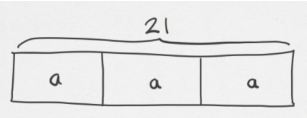Pupils should be able to make links with other work they may have done on bar models and if they are not familiar, the visual representation will still provide clarity for the next steps of their working.

Regardless, further grounding in bar modelling would be of significant benefit for these pupils. For more bar model guidance see ‘How to teach the bar model method to ace arithmetic and word problems’, and ‘Using bar modelling techniques to solve multi-step KS2 SATs problems’.

Note with pupils that there are 3 bars (3 ‘a’s) that total 21, therefore we can divide 21 by 3 to find out the value of ‘a’. Then explain to the pupils that often they will be asked to use that information in another part of the algebra question.

In this instance, they should be able to see they can use this in a + a + b = 25 because there is no ‘a’ in the other line. Then you can represent the second line in another bar model and pupils should see that because they know ‘a’, and there is only 1 other missing value, they can use what they know to work the final missing value.

The equation becomes 25 – 7 = 18. 18 – 7 = 11. At this point, they should be able to understand that ‘b’ must be 11.

The final step of the example would be the same process as the second line, 32 – 11 = 21. 21 – 11 = 10. So ‘c’ must be 10.Now you can continue to give examples of stand-alone algebraic equations where pupils can practice using bar models, being mindful, and look at where they can limit the unknowns using this logic and methodology.

You should repeat examples until pupils can confidently work them out, whilst understanding that:

• Bar models can help make what you need to do clearer.
• They should look for what they know and focus on where they can limit the unknowns to 1 (where possible).
• They should work step by step in a logical order

To get your pupils practising using bar models, download this free resource of 25 scaffolded word problems with and without bar models.

When pupils can do the above, they are ready to move on to the ‘Securing’ stage in algebra.

#### Misconceptions and strategies for ‘Securing’ in algebra

Once pupils are more comfortable with the majority of isolated algebra calculations, it is time to add pupils like Sienna into the mix, with a focus on applying the skills learnt into a range of contexts.

If pupils like Sienna struggle at this stage compared to others who have been through the ‘Developing’ Bootcamp section, continuing through from this point should provide some direction. If it is not enough, however, take them back to the ‘Developing’ section.

Below are 2 examples of the kinds of contextualised algebra that the pupils need to become familiar with. The golden rule with all these examples is to continue to follow the bullet points above and encourage pupils to draw on their existing missing numbers understanding:

##### Contextualised algebra – fractions

From the information below work out, what the value of ‘c’:

1 = a + 2/5
b = 2a + 4a
b ÷ a = c

The only new content here apart from fractions is ‘2a’ and ‘4a’ as a representation of 2 x a and 4 x a. Pupils should be made familiar that this is short-hand for multiplication in algebra.

As with the previous examples, use bar models such as these where needed: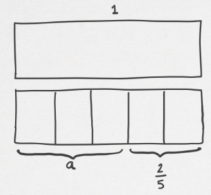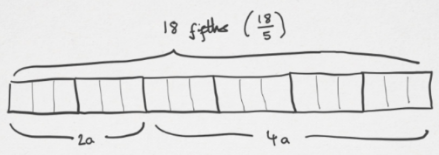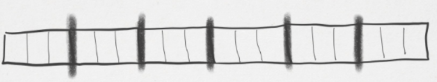Pupils should be calculating ‘a’, then using that to calculate ‘b’ before using both ‘a and b’ to work out c.

Once they are comfortable with this, you could then jumble the letters up and ask the pupils to re-calculate to help them practise with non-consecutive letters.

If pupils struggle it is imperative to work out if the problem is with algebra or with fractions. If the pupils are insecure with the fractions content you provide them with, try our fractions bootcamp.

##### Contextualised algebra –  measures

Formulae are great fun for enquiring minds and – with a few nudges along the way – pupils should be able to transfer their understanding so far to using formulas to solve calculations in different contexts, like this measures question:

The Perimeter of a rectangle is:  n = 2(m + o). If a rectangle has a length of 27cm and a width of 13cm, show your working and apply the formula to find the perimeter of the rectangle.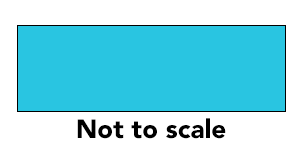The pupils should realise that the process is almost exactly the same as with the previous algebra examples: find what you know & simplify. The difference is in swapping out the unknowns rather than calculating them, i.e. n = 2(27 + 13). So 27 + 13 = 40. 40 x 2 = 80. Therefore the perimeter is 80cm.

A bar model like the one below could help understanding here but, once the pupils gain experience with formulae, their prior experience should help them in all but the most difficult questions.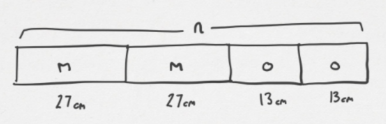Once the pupils can answer these kinds of questions with just the odd prompt, they are ready to tackle the full range of Primary algebra content.

If pupils embed being mindful, looking for what they know and, applying that by limiting the unknowns whilst working in a logical order, they will have their own formula for success with algebra that they can apply to a wide range of contexts and content.

If your pupils have gaps that still need plugging in the run up to SATs, don’t panic! Read our blog on Year 6 Maths SATs preparation.

It has everything you need: free practice SATs papers, testing best practice, and guidance on making sure your pupils feel comfortable, straight from a primary headteacher.

Primary school tuition targeted to the needs of each child and closely following the National Curriculum.##### Pete Richardson
Walton Le Dale Primary School
Pete is a passionate convert to a mastery approach, blending the best of Singapore, Shanghai & home grown pedagogy. He blogs for Third Space on Mastery, & more!

Related Articles

x#### Algebra Lessons Resource Pack

Plug gaps and help conquer common upper KS2 misconceptions in algebra

#### Algebra Lessons Resource PackPlug gaps and help conquer common upper KS2 misconceptions in algebra